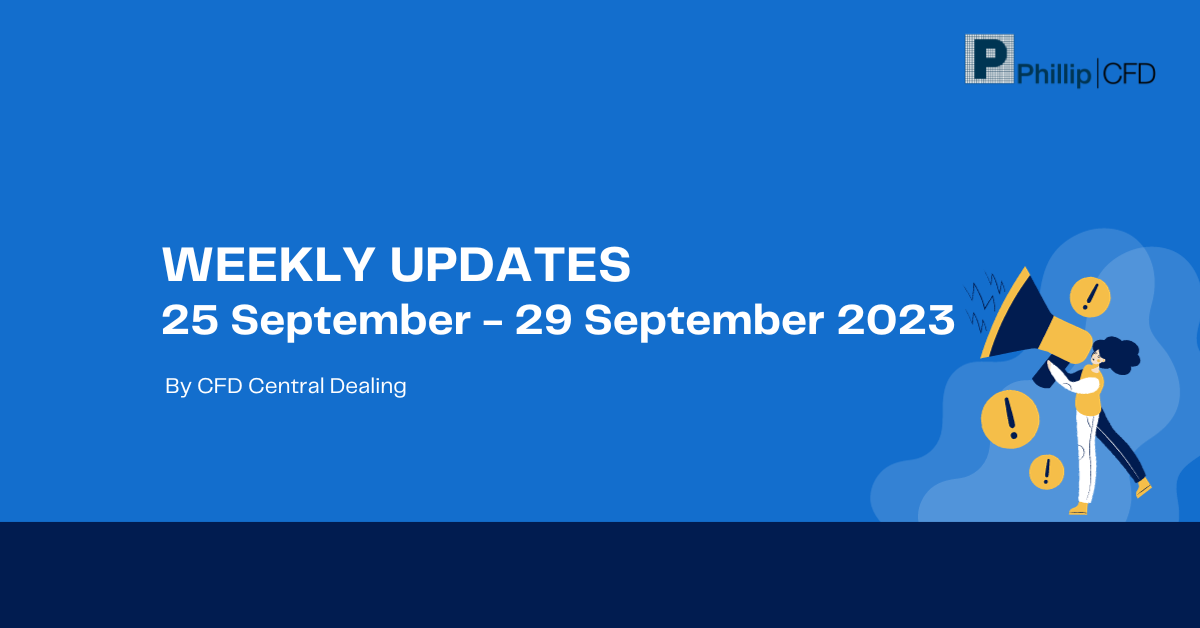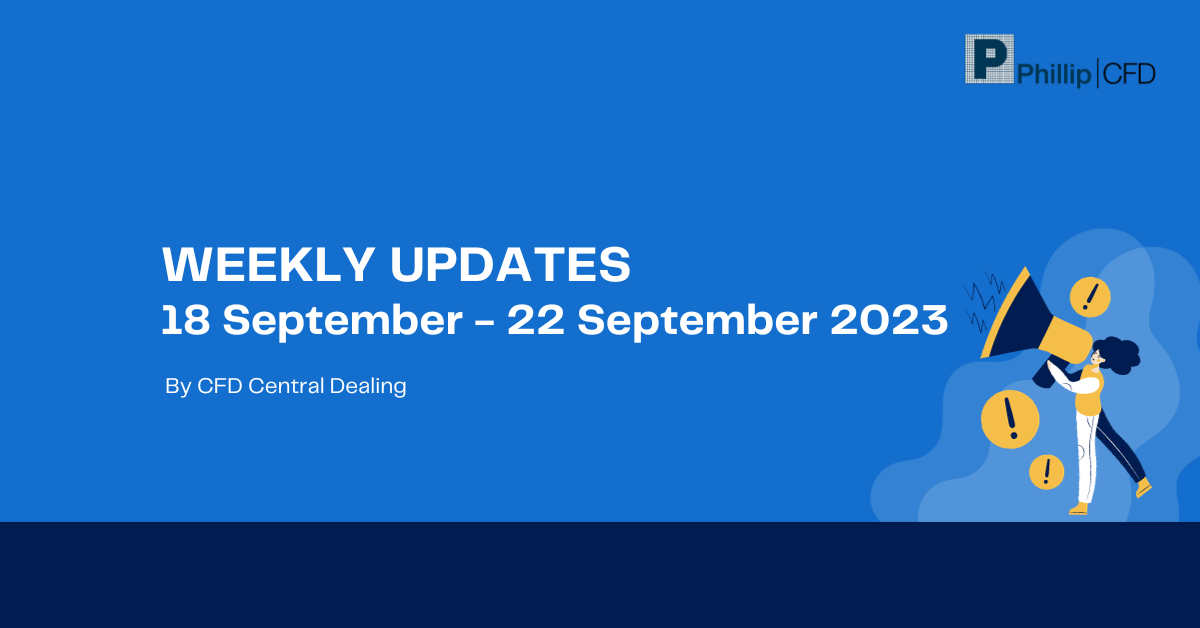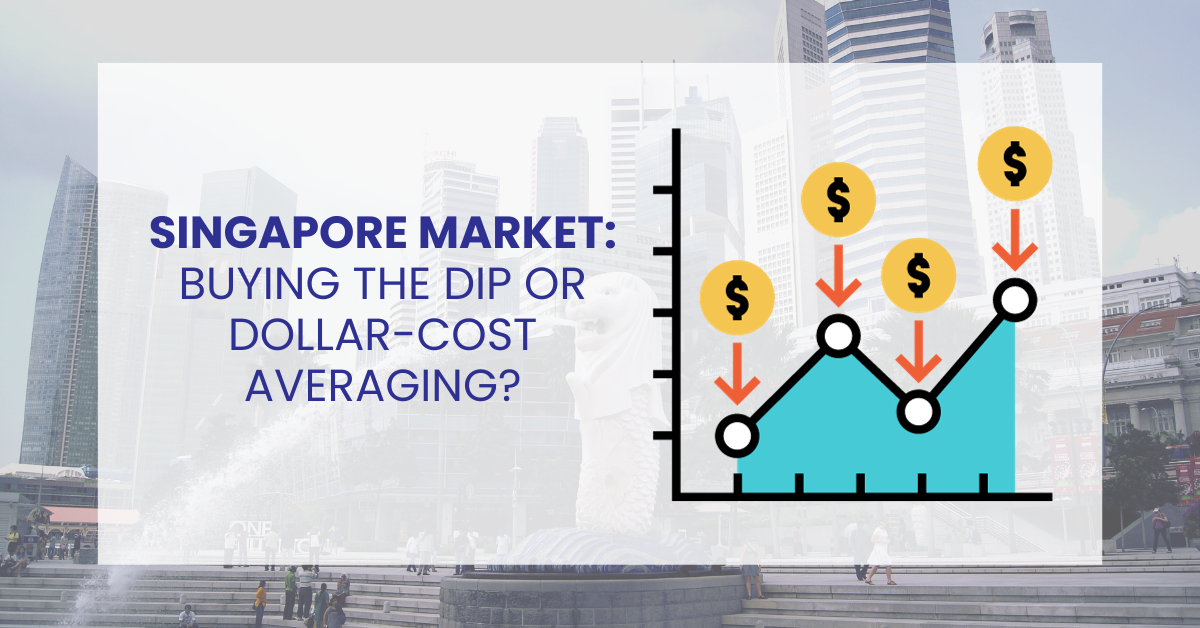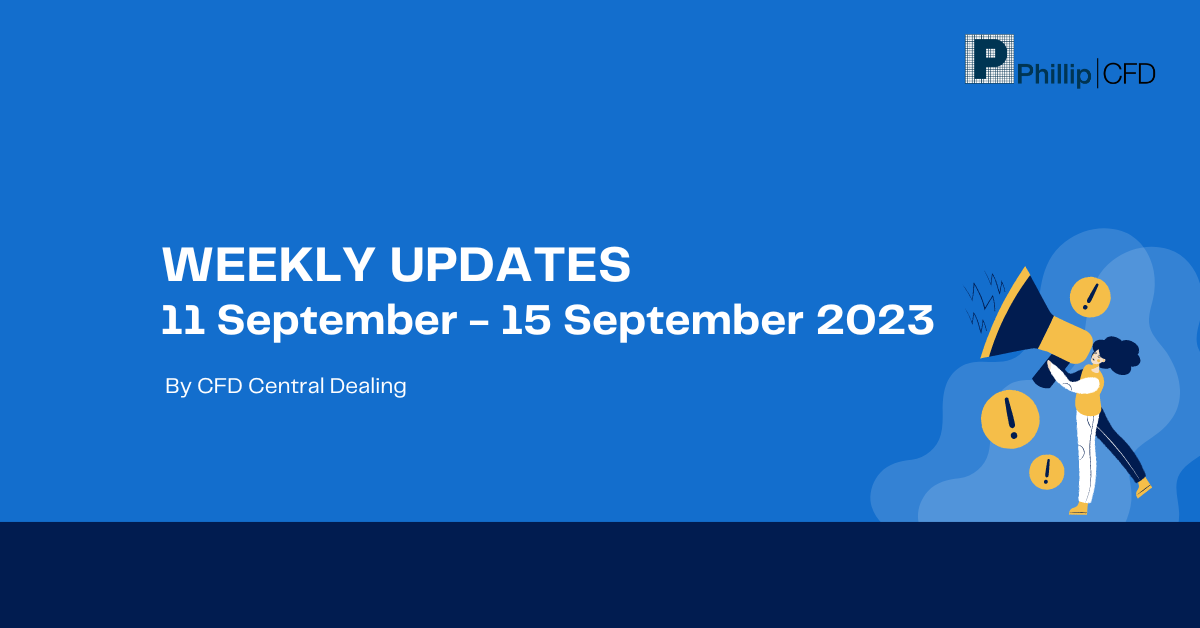﻿ Correlation coefficient:

# Correlation coefficient

## Correlation coefficient

The correlation coefficient is a crucial indicator in statistical analysis, revealing the link between two variables. This statistical measure, which is widely used in domains such as economics, social sciences, and finance, tries to measure the degree of relationship between two elements, providing essential insights into the intensity and direction of their dependency. This formal exposition will dig into the complexities of the correlation coefficient, outlining its fundamental concept, clarifying its calculation process, and elucidating its range and interpretative importance.

## What is correlation coefficient?

The correlation coefficient is a statistical measure that quantifies the degree of association or relationship between two variables. It assesses how changes in one variable relate to changes in another. The correlation coefficient runs from -1 to 1, with -1 denoting a perfect negative connection, 1 denoting a flawless positive correlation, and 0 denoting no association in linear correlation.

Moreover, it sheds light on the limitations inherent in its application, thereby accentuating the importance of exercising prudence and caution while interpreting the outcomes derived from this statistical tool. Thus, in a world of data-driven decision-making, understanding the essence of the correlation coefficient assumes paramount significance, as it arms analysts and researchers alike with the means to discern patterns and anticipate trends with precision and rigour.

## Understanding correlation coefficient

A correlation coefficient is crucial in analysing data and making predictions in various fields, such as economics, social sciences, and finance. It helps researchers and analysts identify the degree and the course of a link between two factors.

Understanding the correlation coefficient is critical for researchers and analysts since it simply assesses the link between two variables. It offers a full knowledge of how changes in one variable may affect the other by determining the intensity and direction of this relationship. The correlation coefficient functions as a compass, leading decision-makers through the maze of data and guiding them to relevant conclusions.

When the correlation coefficient is positive, often between 0 and 1, it indicates a direct relationship between the two variables. A rise in one variable correlates with an increase in the other, and vice versa. A one correlation value indicates that the variables move in the same direction.

However, a correlation coefficient near 0 suggests an absence of any discernible linear relationship between the variables. It neither conveys a clear connection nor implies independence. In such instances, prudence dictates that one ought to look beyond the correlation coefficient and explore the presence of any non-linear relationships or lurking confounding factors.

## Limitations

While the correlation coefficient is a valuable tool, it does have some limitations that should be considered:

• Correlation does not imply causation

A high correlation between two variables does not necessarily mean one causes the other. There may be hidden or confounding variables influencing both.

• Non-linear relationships

As the correlation coefficient only assesses linear correlations, it is useless and might not capture complex, non-linear associations.

• Outliers

Outliers can significantly influence the correlation coefficient, potentially leading to misleading results.

• Restricted to two variables

The correlation coefficient can only measure the relationship between two variables at a time, not considering potential interactions with other variables.

## Examples

Example 1

We want to see if there is a link between the number of hours studied and the test results of a set of pupils. We discovered that the correlation coefficient is 0.75 after gathering data. This positive result indicates a somewhat favourable association, indicating that students who study are more likely to perform better on tests.

Example 2

Let’s consider the relationship between rainfall and crop yield. A correlation coefficient of -0.6 is obtained, indicating a moderate negative correlation. This suggests that as rainfall increases, crop yield tends to decrease, and vice versa.

To calculate the correlation coefficient between two variables, follow these steps:

• Calculate the means of the two variables.
• Subtract the mean of each variable from its respective data points.
• Multiply the differences for each data point and sum the products.
• Calculate the sum of squares for both variables.
• Divide the sum of products (from step 3) by the square root of the product of the sum of squares (from step 4) of both variables.

The correlation coefficient ranges from -1 to 1. A value of -1 represents a perfect negative correlation, 1 indicates a perfect positive correlation, and 0 denotes no linear correlation.

Positive correlation refers to a relationship between two variables where an increase in one variable is associated with an increase in the other. A positive correlation coefficient (between 0 and 1) indicates the strength of this relationship.

To calculate the correlation coefficient between two variables, compute the covariance of the paired data points and normalise it by the product of their standard deviations.

Subtract the mean from each data point, multiply the resulting variances for both variables, and add them. Then, for each variable, compute the squared deviations and aggregate them individually.

Finally, divide the covariance by the square root of the sum of the squared deviations for both variables. The correlation coefficient, which might range from -1 to +1, will be the outcome. A positive number implies a positive linear association, a negative value shows an inverse relationship, and a value approaching zero indicates a weak correlation.

To determine if a correlation is positive or negative, examine the sign of the correlation coefficient. If the coefficient is positive, it indicates a positive correlation; if it is negative, it indicates a negative correlation.

## Category

### Read the Latest Market Journal#### Weekly Updates 25/9/23 – 29/9/23

Published on Sep 25, 2023 27

This weekly update is designed to help you stay informed and relate economic and company...#### Top traded counters in August 2023

Published on Sep 19, 2023 276#### Weekly Updates 18/9/23 – 22/9/23

Published on Sep 18, 2023 33

This weekly update is designed to help you stay informed and relate economic and company...#### The Merits of Dollar Cost Averaging

Published on Sep 15, 2023 56

Have you ever seen your colleagues, friends or family members on the phone with their...#### Singapore Market: Buy the Dip or Dollar-Cost Averaging?

Published on Sep 14, 2023 48

To the uninitiated, investing in the stock market can be deemed exhilarating and challenging. The...#### What are covered calls and why are they so popular?

Published on Sep 12, 2023 560Published on Sep 11, 2023 33